### A LEGO ® MINDSTORMS® EV3 Polygon Gyrotracker

#### March 28, 2017   mindstormsEV3STEMpolygonsgyro sensor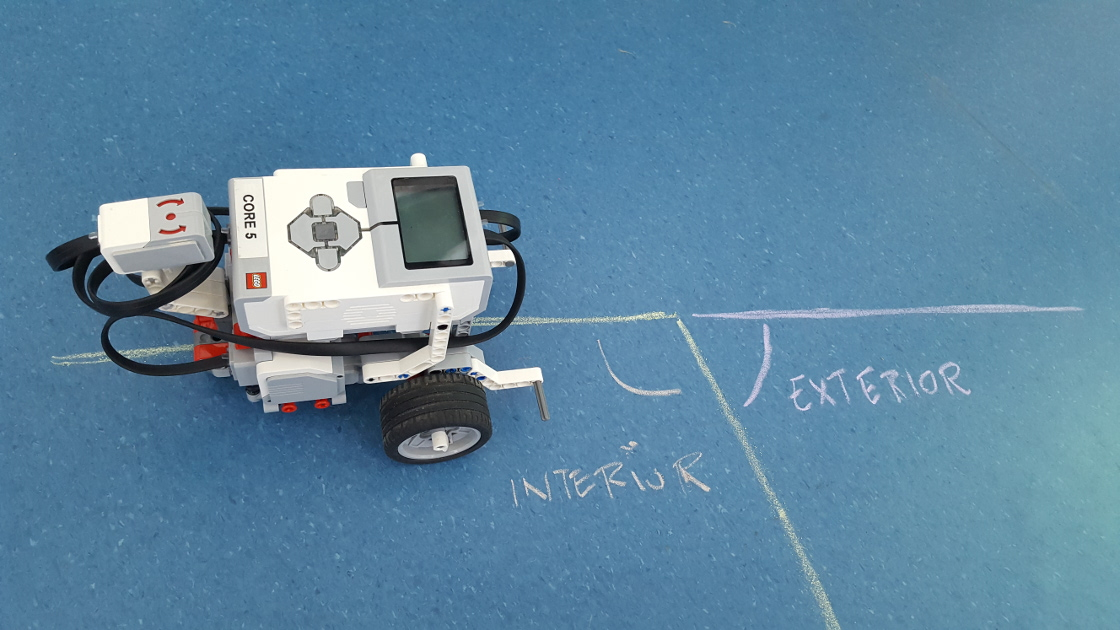# A LEGO ® MINDSTORMS® EV3 Polygon Gyrotracker

In this lesson we’ll learn about regular polygons, what is an exterior angle and what’s the relationship between the number sides of a regular polygon and it’s exterior angle.

We’re using the Robot Educator Model, equipped with the Gyro Sensor to progressively understand this concepts and build a fully functional model that can track any regular polygon taking the number of sides as an input.

At the end of this lesson, our students will have a solid knowledge of:

• What’s a regular polygon.
• What’s an exterior angle.
• Calculating the exterior angle for a regular polygon
• Using the Move Steering, Gyro Sensor, Math, Loop and Wait (for Gyro Sensor and Brick Buttons) blocks, as well using variables.

Regular polygons are polygons on which all angles are equal in measure and all sides have the same length, such as triangles, squares, pentagons, hexagons and so forth.

Let’s first make sure that the students are familiar with these polygons by making them fill a table like shown in the screenshot below. Ignore the exterior angle column for now; we’ll deal with in the next task.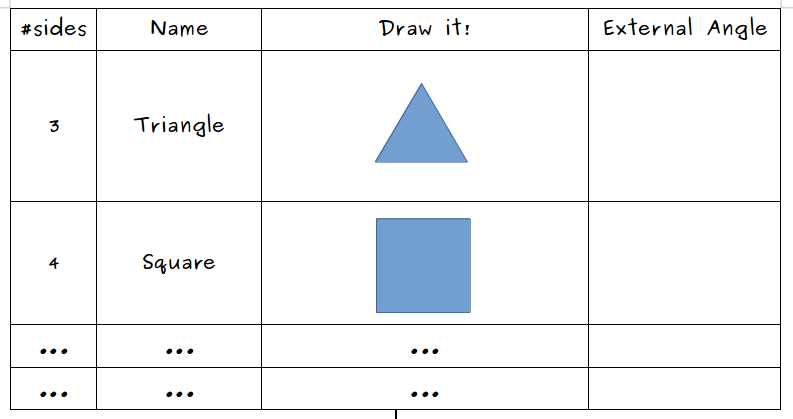Once the students have completed the table with the polygons from the triangle to the hexagon it’s time to move onto the exterior angle.

As per this (somewhat obscure) definition,

The Exterior Angle is the angle between any side of a shape, and a line extended from the next side.

Let’s shed some light on this by taking a look at the diagram below, where we can see that the interior angle is part of arc filled in blue, whereas the exterior angle is the one filled in white: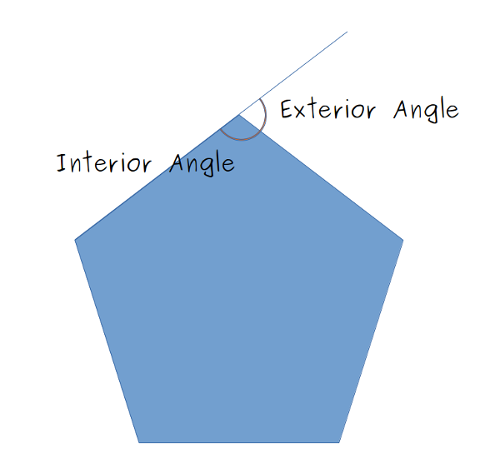Before moving forward, let’s pause for a second and make a few experiments involving these angles:

1. Calculate the sum of the interior and exterior angles. They add up to 180°, hence they are supplementary angles.
2. Now try this: draw a polygon with chalk on the floor, for instance, a square. Put the Robot Educator on one of the corners and push it along the sides. Note how much you turn on each corner… Yes: it’s the exterior angle!
3. Go round the square along the sides, turning on all corners until completing one full loop. You should reach the starting point facing the same direction, which means that all the turns you made add up to a full 360° turn, that is: `exterior angle * number of turns = 360`.

Therefore, the exterior angle for a regular polygon can be calculated as:

``````exterior angle = 360 / number of corners
``````

Now that we know how to calculate it, go back to table on the previous task and fill in the exterior (or external) angle for the polygons, before moving into coding.Based on all the facts that we’ve collected about interior and exterior angles in a polygon it should be fairly simple to come up with a similar formula for calculating a regular polygon’s interior angle.

## Task 3: Hardcoded triangle and hexagon

Now that we know what’s the value of the exterior angle for a polygon let’s program our Robot Educator for tracking the shape of a triangle and an hexagon using the gyro sensor.

Here’s the program for the triangle: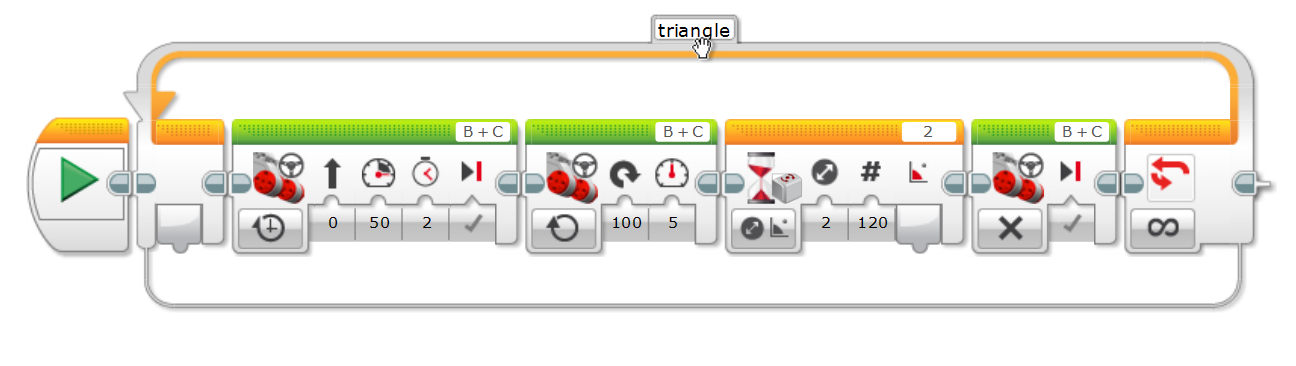And for the hexagon: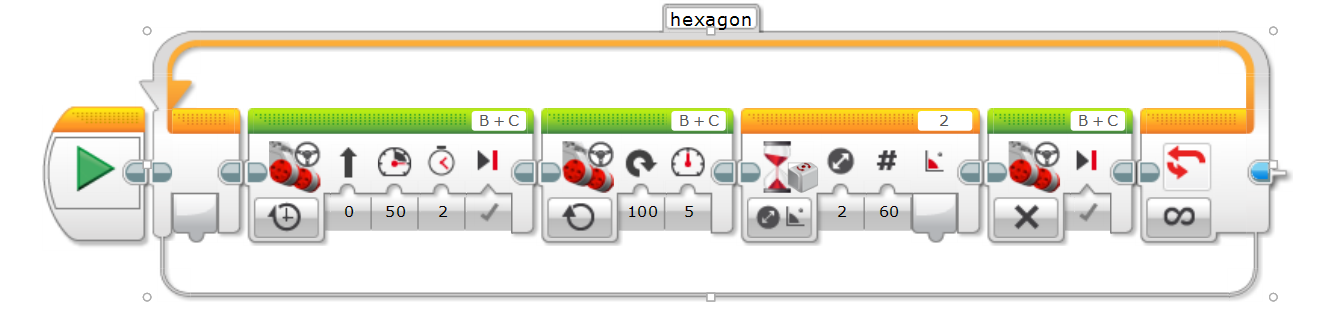Both programs are almost identical and repeat in an endless loop:

• Move forward for 2 seconds.
• Turn right until the angle changes by the exterior angle. Let’s use the values we’ve previously written down in the table: 120° for triangle and 60° for the hexagon.

Keep in mind that the gyro sensor reading lags a little bit, hence the robot will turn a few extra degrees on each corner. A proper way to compensate this lag is outside the scope of this lesson, for now we’re minimizing the impact by turning real slow (power 5).

This program is the basic flow we’ll reuse in the following tasks.

## Task 4: Computed Exterior AngleDid you notice the sole sole difference between the previous two programs?The value of the exterior angle.

Let’s reuse the basic flow by calculating the exterior angle given the number of corners in the polygon as shown on the program below: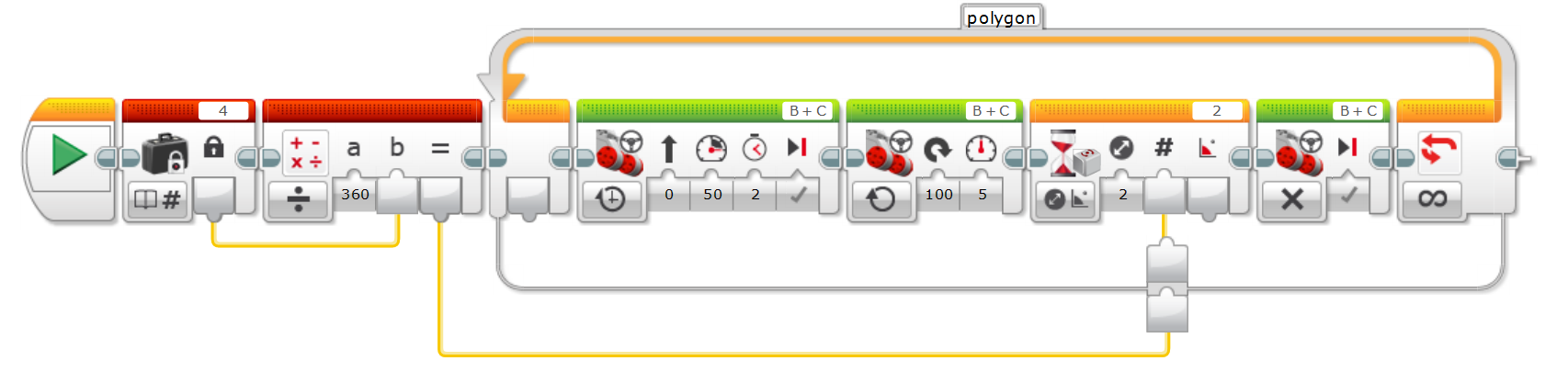In this program we’re:

• Defining a constant representing the number of corners in the polygon
• Passing the constant to a Math Block which calculates the exterior angle applying the formula `exterior angle = 360 / number of corners`
• Wiring the output of the Math Block to the input of the Wait Block.

Now that we know how to compute the exterior angle, let’s make our program a little bit more flexible by using variables.

## Task 5: Displaying the corners variables

In the previous task we used a constant for specifying the number of corners in the polygon. This time we’re going to use a variable for storing it.

For debugging purposes and in order to simplify the flow we’ll use a secondary program to display the value of the variable on screen throughout the main program execution.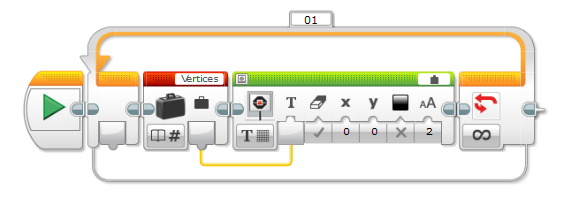## Task 6: User Defined Polygon

Now that we have a variable holding the number of corners, it’s time to allow the user to input it’s value: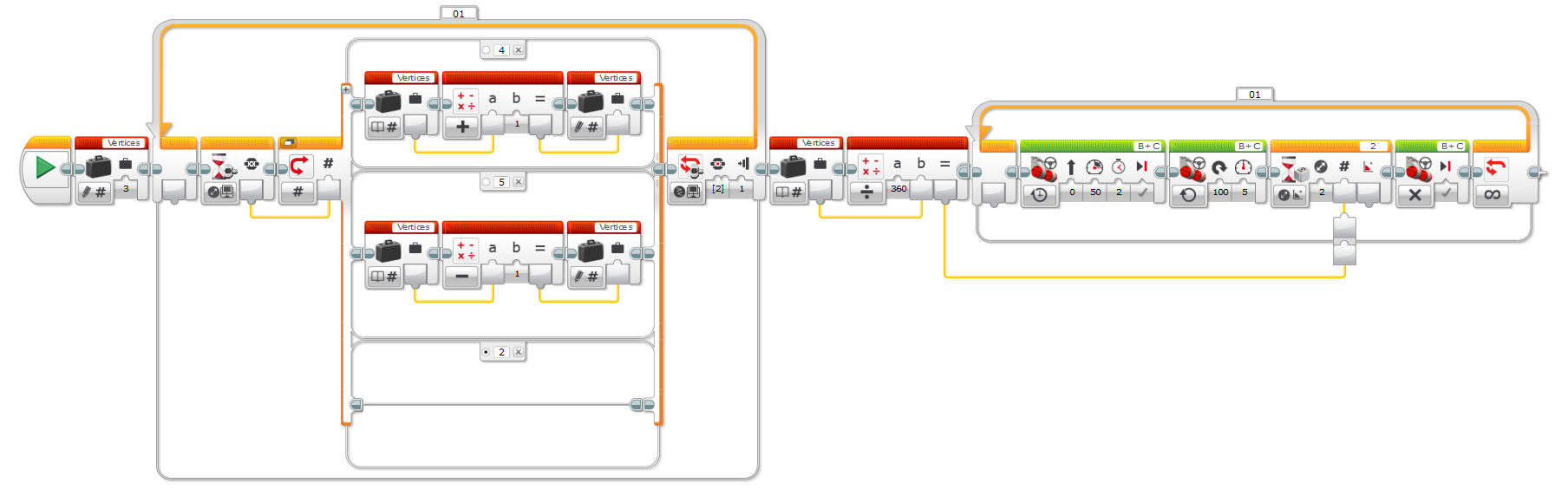In this program we’re:

• Setting the initial value of the variable to 3, which is the minimum number of corners in a polygon.
• Waiting for the user to press a Brick Button.
• Passing the value of the pressed button to a Switch Block which:
• Increments the variable when pressing the Up (4) button.
• Decrements the variable when pressing the Down (5) button.
• Does nothing on the default case.
• The loop exits when the pressing the Center (2) button.
• The variable is then fed to the match block which calculates the exterior angle.One of the questions that usually pops up is: why are we adding all this complexity to the program when we could perfectly live with hardcoding the value in the constant?We can answer that question with another question: Let’s say you programmed your Robot Educator for tracking a square. How would you track an hexagon if you didn’t have your programming environment handy? By making the program runtime configurable we give it more flexibility, at the expense of simplicity.The minimum number of corners in a polygon is 3. Can you modify the program so that the variable can’t be set below this minimum value?

## Task 7: Full Polygon Gyro Tracker

Our program is almost complete now, but you’d probably noticed that once we provide the number of corners, the program will run forever tracking the specified polygon. This is nice, but we’d rather track one full polygon and then prompt the user again for providing a new number of corners.

This can be achieved by wiring the corners variable to the exit condition on the basic flow, and wrapping the previous program in an endless loop, as displayed on: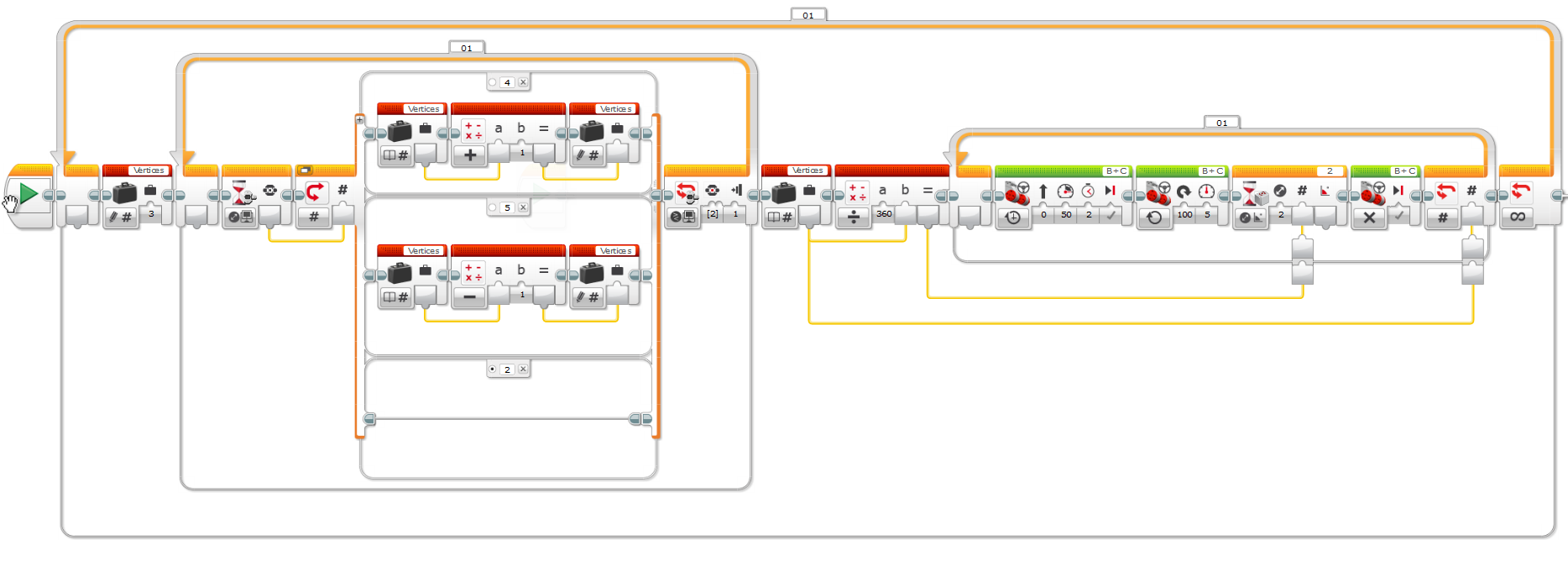## Conclusion

In this lesson we learned how to build a somewhat complex program with user input and some maths that helped us better understanding some interesting facts about regular polygons.